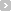#### margin和profit都表示利润，有什么区别？2013/09/24| |by karasantoso

Margin is used to compare A relative to B, where A is part of B. Margin is usually stated as a percentage.

Followings are two examples of margins:

1. Gross profit margin = Gross profit / revenue;
2. Net profit margin = Earning after tax / revenue;

In accounting, a simple expression of a profit and loss statement is as follows:

Revenue 1,000
Cost of good sold -900
Gross PROFIT 100
Operating expenses -10
other expenses -2
tax -3
net PROFIT after tax 85

then, Gross profit MARGIN = 100/1000 = 10%.
Net profit MARGIN = 85/1000 = 8.5%

--------------
PROFIT is a certain amount of benefit obtained from operations of a company or from trading activities. Different from a margin, profit is stated in a full monetary value. In the above example, the gross profit MARGIN is 10% but the Gross PROFIT is 100; and net profit MARGIN is 8.5% but the Net PROFIT after tax is 85.

Hope this can help.加入收藏！美国英语中的"law","bill","regulation"以及"legislation"有什么重要的区别fill in和fill out有什么区别？Jaguar和美洲虎payroll和payslip的区别
revenue 收入，也就是毛收入，比income 正式，一般是国家财政收入
profit 利润，就是去掉本钱后的纯获利
turnover 产值，一般是一年的总产值
margin 边际收益，指增加的部分
income 一般是个人收入